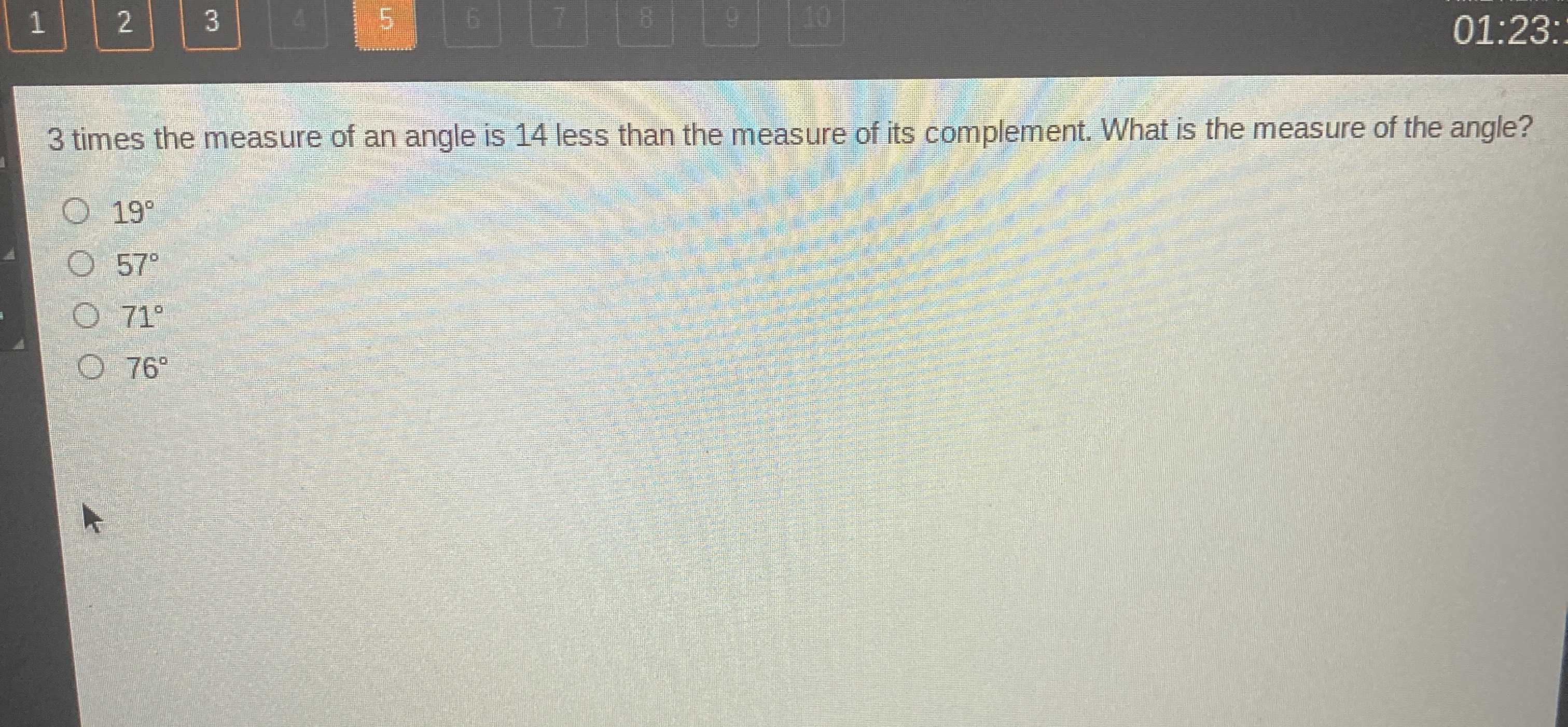### ¿Todavía tienes preguntas de matemáticas?

Pregunte a nuestros tutores expertos
Algebra
Pregunta$$3$$ times the measure of an angle is $$14$$ less than the measure of its complement. What is the measure of the angle?

A. $$19 ^ { \circ }$$

B. $$57 ^ { \circ }$$

C. $$71 ^ { \circ }$$

D. $$76 ^ { \circ }$$